# Metasurface Antenna Modeling

This example shows how to design, model, and analyze a metasurface antenna based on reference . Metasurface antennas take advantage of the periodic boundary of each radiation unit cell to group the radiation unit in a more compact space.

You construct an unit radiation element with Antenna Toolbox™ objects and functions. You also use `infiniteArray` and `rectangularArray` objects to construct the large antenna array, on which you perform pattern and current analysis at the designed frequency.

The unit radiator in  is a cross I-beam structure with an optimized probe feed in one arm. The unit cell has a dimension of 3 cm and gap of 0.5 mm. Specify the geometry of the antenna.

```length = 14.75*1e-3; width = 3*1e-3; s = 0.25*1e-3; thickness = 1.5*1e-3; viaDia = 0.5*1e-3*2; feedWidth = viaDia/2; gndLength = length + 2*s; gndWidth = gndLength;```

Use the `customAntennaGeometry` object to construct the top cross I-beam radiator and place it into a reflector to form the unit radiator structure. In this simplified geometry, you remove the substrate from the design. Removing the substrate increases the radiation frequency to 9.14 GHz, which is higher than the value in .

```s1 = antenna.Rectangle(Length=length, Width=width); s2 = antenna.Rectangle(Length=width, Width=length); s3 = antenna.Rectangle(Length=feedWidth, Width=feedWidth); [~] = translate(s3,[-5*1e-3+feedWidth/2 0 0]); pr1 = getShapeVertices(s1); pr2 = getShapeVertices(s2); pr3 = getShapeVertices(s3); radiator = customAntennaGeometry(Boundary={pr1,pr2,pr3}, Operation="P1+P2+P3"); radiator.FeedLocation = [-5*1e-3 0 0]; radiator.FeedWidth = feedWidth; ant = reflector(Exciter=radiator, GroundPlaneLength=gndLength, GroundPlaneWidth=gndWidth, ... Spacing=thickness, EnableProbeFeed=true); figure show(ant)```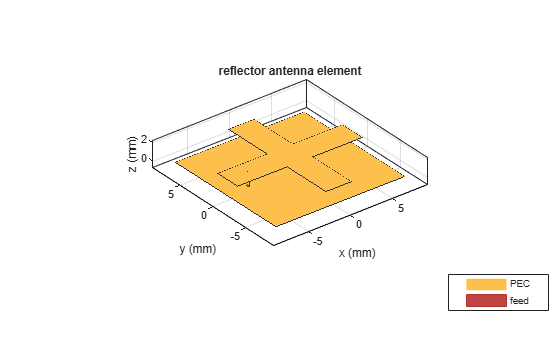### Mesh and Analyze Unit Structure

Generate a mesh with a maximum edge length of 0.1 m.

```figure; mesh(ant,MaxEdgeLength=0.1);```Calculate the impedance as a function of frequency.

```freq = 9.14e9; figure; impedance(ant,freq*[0.8:0.01:1.1]);```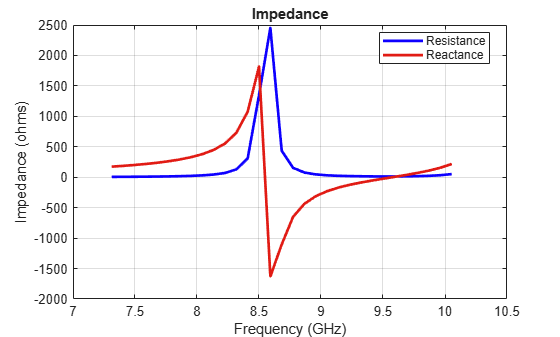### Implement Periodic Boundary and Analyze Antenna

To include the periodic boundary effect on the unit radiator, specify the radiator as the element in the `infiniteArray` object. The object constructs an infinite antenna array with the designed radiator. Numerically, the object uses a Green function with a method of moments (MOM) and models the coupling effect between adjacent unit radiators.

```infArray = infiniteArray(Element=ant); figure show(infArray)```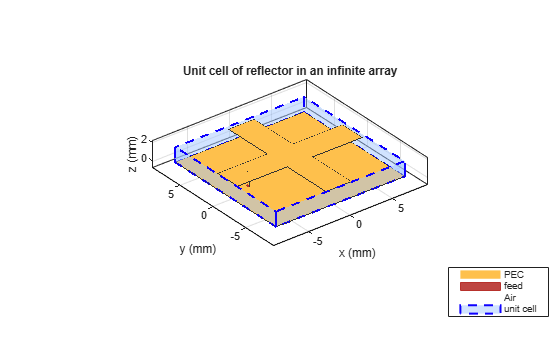```figure current(infArray,freq)```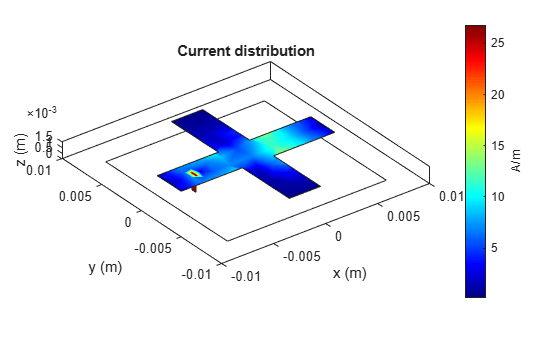### Analyze Finite Array

To analyze the coupling effect on a finite array, construct a 5-by-5 element rectangular array with the designed radiator.

```array = rectangularArray(Element=ant); array.Size = [5 5]; array.RowSpacing = gndLength; array.ColumnSpacing = gndWidth; figure show(array)```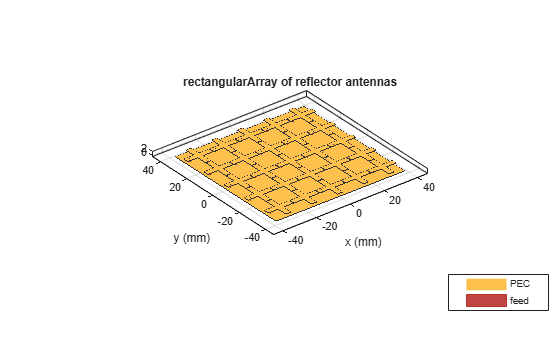Plot the pattern and current of the array as functions of the frequency.

```figure pattern(array,freq)```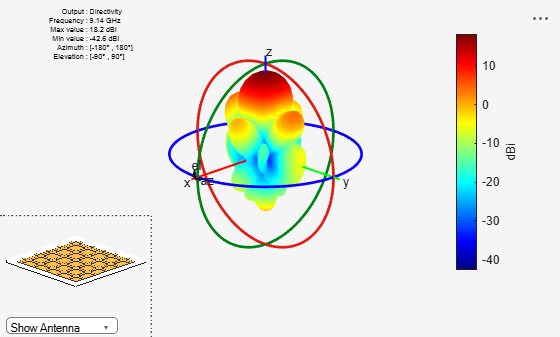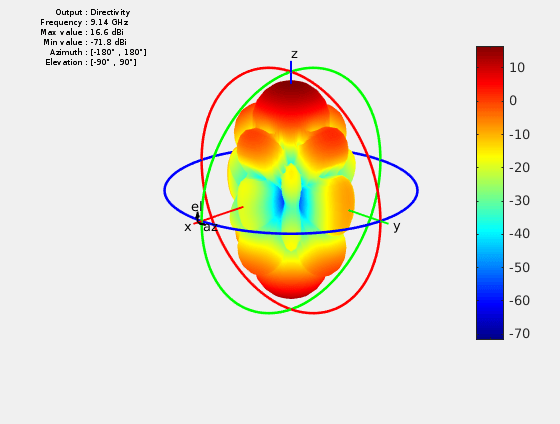```figure current(array,freq)```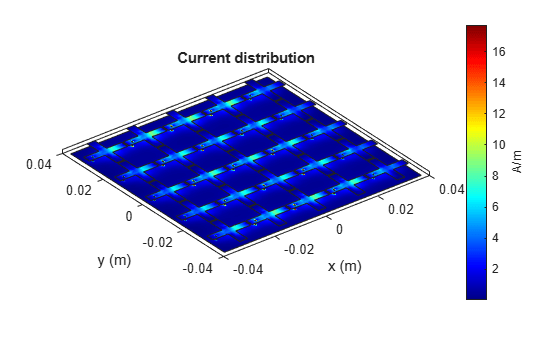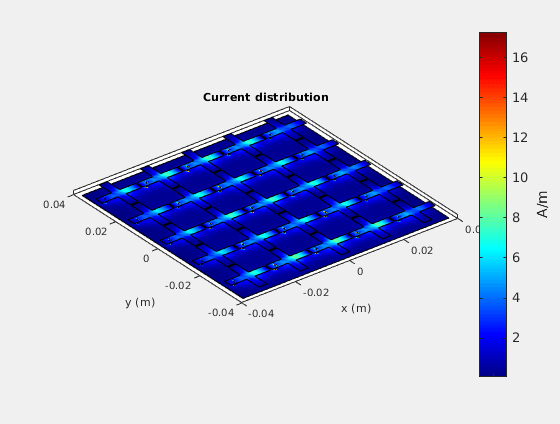### Assign Substrate to Infinite Array

Specify Teflon™ as the substrate of the unit cell element and visualize the geometry of the infinite array.

```infArray.Element.Substrate = dielectric("Teflon"); figure show(infArray) ```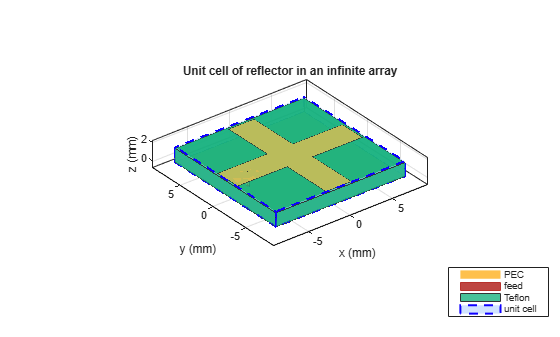### Mesh Unit Cell

Mesh the unit cell, specifying a maximum edge length of `lambda/40,` where lambda is the free-space wavelength at 9.14 GHz. The software captures the dielectric effect in the infinite array analysis in the equivalent periodic Green's function, so use the MOM over the top layer metal mesh only.

```lambda = 3e8/9.14e9; figure mesh(infArray,MaxEdgeLength=lambda/40) ```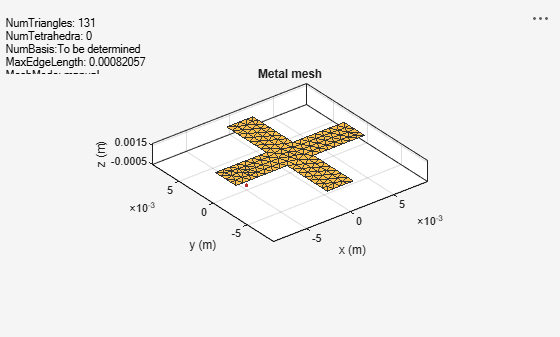### Compute Pattern

With the meshed geometry, perform the full-wave radiation pattern analysis at 9.14 GHz.

```figure pattern(infArray,9.14e9);```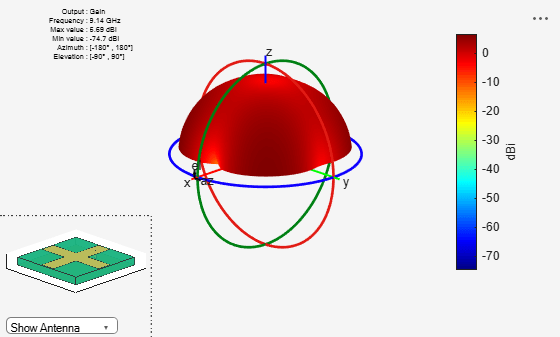### Conclusion

The current and pattern modeling result obtained from the finite array are qualitatively similar to the results reported in . For other types of metasurface geometry, you can construct the unit cell by using an antenna catalog element, as the Infinite Array of Microstrip Patch Antenna on Teflon Substrate example shows, or using a PCB stack.

### References

 Badawe, Mohamed El, Thamer S. Almoneef, and Omar M. Ramahi. “A True Metasurface Antenna.” Scientific Reports 6, no. 1 (January 13, 2016): 19268. https://doi.org/10.1038/srep19268.